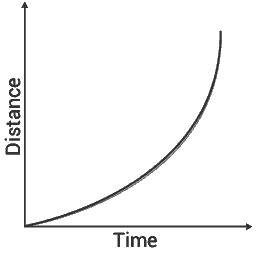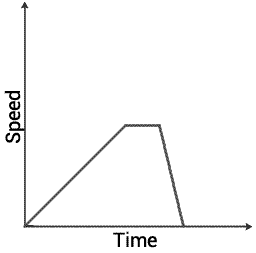# OCR Gateway B Module P3: Forces for Transport

## Speed:

• `Average speed (metres per second - m/s) = distance (metres) ÷ time (seconds)`
• Instantaneous speed is the speed at a certain point

## Distance-time graphs:

• Distance-time graphs show the total speed travelled so far at a certain time
• Here is an example. The speed is constantly increasing (accelerating)• The gradient is found by taking two points on a line, and dividing the `change in y by the change in x`
• This gradient shows the speed, in m/s
• If a line is straight, speed is constant. If it is changing, the line will be curved

## Acceleration:

• `Acceleration (m/s2) = change in speed (m/s) ÷ time taken (s)`. If the result is negative, then it is decelerating (slowing down)

## Speed-time graphs:

• Speed time graphs show the speed at a given point in time
• The following graph could show a short car journey. The car starts accelerating, remains at a constant speed for a while, then quickly decelerates to stationary (not moving)• Acceleration is the gradient of a speed-time graph
• The area under the line(s) shows the distance travelled

## Relative velocity:

• Velocity is a vector, because it has both a speed and direction
• If two cars drive in opposite directions, each at a velocity of 10m/s, then the relative velocity is 20 m/s; the velocities of the two objects are added (10 + 10 = 20)
• If two cars drive in the same direction, each at a velocity of 10m/s, then the relative velocity is 0 m/s; the smallest velocity is subtracted from the largest (10 - 10 = 0)

## Circular motion:

• If a car is moving around a roundabout at a constant speed, such as 10 m/s, it is accelerating because it's not going in a straight line; acceleration is directed to the centre of the roundabout in order to turn the car. If this force stops being applied, the car will continue in a straight line

## Force:

• `Force (Newtons - N) = mass (kg) x acceleration (m/s2)`

## Thinking and braking distance in motor vehicles:

• Humans have a reaction time, the time it takes after something happens for them to take action upon it
• Reaction time increases if the driver is tired, under the influence of drugs (including alcohol), or distracted
• If the vehicle is moving, it will travel a certain distance whilst the driver is reacting, this is called the thinking distance. At higher speeds, reaction time remains the same but thinking distance increases because more distance has been travelled in the same time
• At higher speeds, braking distance is higher. Braking distance is the distance the vehicle travels once brakes have been applied before it completely stops
• Braking distance increases if the road conditions are poor (icy/wet), if brakes are worn (which would reduce the friction between brake pads and the discs), or if the tires have little tread, especially on a slippery road
• When speed doubles, braking distance quadruples

## Work:

• `Work done (Joules - J) = force (N) x distance moved in the direction of the force (m)`

## Weight:

• Weight is the gravitational attraction of an object towards the centre of a body, like the Earth (the downwards force)
• `Weight (N) = mass (kg) x gravitational field strength (N/kg)`. The gravitational field strength of the Earth is about 10 N/kg

## Power:

• Power is the rate in which work is done
• `Power (Watts - W) = work done (J) ÷ time taken (s)`
• This can be combined with the work done formula to get `power = (force x distance) ÷ time taken`

## Kinetic energy:

• `Kinetic energy = 0.5 x mass (kg) x velocity2 (m/s)`
• When a car stops, all its kinetic energy changes into other forms of energy, mainly heat in the tyres, brakes and road
• `Braking force x braking distance = loss in kinetic energy`

## Factors affecting fuel consumption:

• Amount of energy required to increase its kinetic energy
• Speed
• How it is driven (driving at a constant speed is more fuel efficient)
• Energy required for it to move; more friction between the car and the road and the car and air will require more energy

## Electric cars:

• Issues with electric cars:
- Batteries take up lots of room and can be heavy
- They are expensive to produce and buy
- Many have a smaller range than a conventional fossil-fuel powered car
- Solar powered cars rely on the sun shining and backup batteries
• Recharging uses electricity, which is often produced using fossil fuels
• Biofuels may reduce carbon dioxide emissions because plants which are grown to produce it absorb it for photosynthesis. However, trees are being chopped down to get land to grow biomass, so this is not certain

## Force and momentum:

• `Momentum (kg m/s) = mass (kg) x velocity (m/s)`
• Spreading momentum change in a car crash over a long period of time reduces the force (`change in momentum ÷ time`)
• Force can be decreased by reducing the acceleration, by increasing collision time or distance
• Many car safety features, such as seat belts, air bags, crash barriers, crumple zones and escape lanes all achieve this

## Car safety features:

• Modern cars absorb energy when a vehicle suddenly stops; brakes get hot, crumple zones change shape, seat belts stretch (and convert kinetic energy to elastic energy) and air bags inflate
• Some people don't wear seat belts because there is a risk of chest injury, there is a risk of being trapped if there is a fire, and they encourage the driver to drive more carefully
• Seat belts have to be replaced after a crash because the large forces may damage them
• ABS (anti-lock braking system) brakes prevent the wheel from locking up and uncontrollably skidding when braking by removing and reapplying brakes repeatedly. They may also reduce braking distances
• Wheel speed sensors record the rotational speed, and a computer controls pressure to brakes, increasing braking force just before the wheels stop to skid
• Electric windows (rather than manually winding them up/down) and paddle shift controls to change gear allow the driver to concentrate on the road
• Cruise control stops a driver getting tired and pressing harder on the accelerator

## Falling objects:

• If there is little air resistance, all objects fall at the same acceleration
• Gravity is usually greater than air resistance, so the object falls, accelerating
• If air resistance equals the weight of the parachutist, a terminal velocity is reached and they stop accelerating

## Gravitational field strength:

• Gravitational field strength varies at different points on the Earth's surface and at different heights/depths

## Gravitational potential energy:

• All objects above the ground have gravitational potential energy (GPE), it is converted to kinetic energy as the object falls
• They have GPE because of their mass and position in Earth's gravitational field
• When terminal speed is reached, GPE is lost as it is used to work against air resistance
• `GPE = mass (in g) x gravitational field strength (about 10 m/s2) x height (in m)`
• `GPE = 0.5 x mass x velocity2`, rearranges to `height = velocity2 ÷ 2 x gravitational field strength`

## On a rollercoaster:

• A motor hauls the train high up. At the top, the train has lots of GPE. When it is released and starts falling, it converts to kinetic energy (KE)
• If mass doubles, KE doubles
• If speed doubles, KE quadruples
• `GPE at top = KE at bottom + energy transferred due to friction`